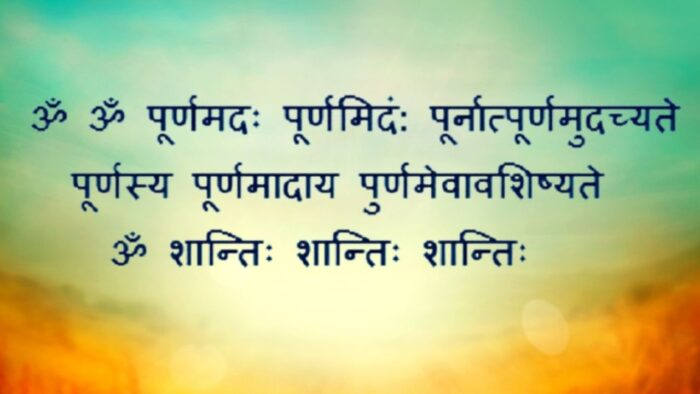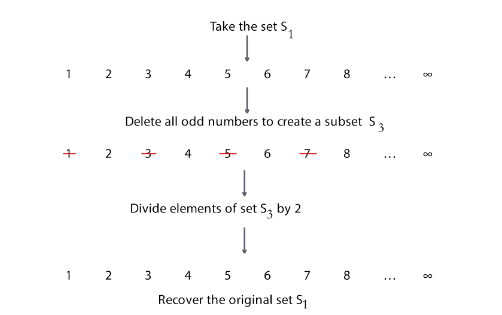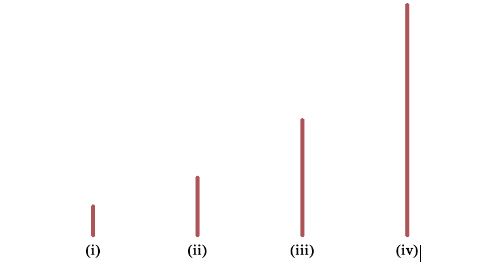# The Infinity Mantra – Part Iर्णस्य पूर्णमादाय पूर्णमेवावशिष्यते- declares the second line of one of the Shanti Mantra(1). This Shanti Mantra is associated with the Svetasvatara (2) and Isha Upanishads (3). In English, the mantra translates to: from the poorna (पूर्ण) when you take out poorna, you are left with poorna (1-3). Poorna is a Sanskrit word, which is usually roughly translated as ‘whole’. In the context of the Shanti Mantra, translators (2,3) have interpreted poorna to be a synonym for Brahman (ब्रह्मन्) and the statement to imply that “Brahman is still full, although the whole universe has come out of it”(2).

## Poorna from Poorna: Is that possible?

To understand this mantra in more detail, let us pretend not know a priori what poorna implies. Then it would seem that the mantra asserts that there is an entity called ‘poorna‘, which allows you to subtract poorna from poorna, and still be left with poorna. This assertion defies common sense, since if it were possible, we probably would all wish to endow our bank accounts with this property.

So, let us do a reality check on this mantra and put this shloka through a rigorous test. Posing this issue in an algebraic manner, we can translate the shloka into the following equation: ?-? = ?, where ? is the entity to be determined. The Shanti Mantra declares that there exists an entity called poorna that fulfills ‘?’ in this algebraic equation. Keeping with our pretense of not knowing what poorna implies, at this stage the variable is an entity that needs to be discovered.

Having taken a daring first step and reduced the Shanti Mantra to its mathematical equivalent, the apparent question is whether entity ‘?’ actually exists. The first and obvious solution is shoonya (शून्य), or zero, which satisfies the above equation by its very definition. But, are there other mathematical solutions that could satisfy the equation?

Let us now investigate the properties of the set of natural numbers, S1 = {1,2,3…..∞} and see if they can be of any assistance to us. Set S1 is an example of a countably infinite set(4) defined as having an infinite number of countable elements, which, even if the counting takes forever, will arrive at a particular element in a finite amount of time. Using set S1, we take the set of natural numbers and delete all odd numbers to create set S3. This is equivalent to subtracting from S1 the set S2 = {1,3,5, … , ∞} to create another set S3.

Set S3 now contains all even numbers i.e. S3 = {2,4,6 … , ∞}. At this point one might intuit that set S3 is approximately ‘half an infinity’, if there is such a thing. But take a closer look at S3 and you will see that it looks very similar to set S1, only the corresponding elements are doubled. In fact, you can divide each element of S3 by ‘2’ and you will recover set S1. The entire operation is depicted in the figure below:To express this in a more rigorous mathematical statement, we say that sets S3 and S1 are both countably infinite and are actually ‘equivalent’. In fact, it can be shown that the set we subtracted (S2) from the original set (S1), also is equivalent to the two sets. This is mathematically denoted as S1~S2~S3, where the symbol ‘~’ denotes equivalence. The above is not a formal proof, but a more rigorous proof for the same can be validated using methods of mathematical analysis (4).

Voila! We took an infinite set and deleted half of that set and we find that the deleted set and remainder are both the same as the set we started out with. Roughly speaking we took ananta (अनंत; meaning – infinity) from ananta and were left with ananta. This weird, or non-intuitive behavior, occurs because the sets are infinite and could not have occurred if the sets were finite. In a strict mathematical sense, we cannot write ∞ − ∞ = ∞, but rather we can say that certain infinite sets seem to satisfy the enigmatic philosophy of the Shanti Mantra. As this mantra is reflective of the paradoxical properties of infinity, let us name it the Infinity Mantra.

The above example used a countable infinite set. Similar paradoxes are possible with uncountable infinite sets. The spirit of this idea is exemplified in the image below, which can be seen from two different viewpoints. From the perspective of physical expression you can view the diagram as four line segments of varying length. From the perspective of points, you can see the four lines as being equivalent sets of uncountable infinite points. At the risk of offending some mathematicians, we can say that the same infinity represents itself as different physical entities.Strictly speaking, infinity is not a number, and infinite sets are known to have paradoxical properties. Interestingly, the same paradoxical notion found in the Infinity mantra of yore, has been visited by mathematicians in recent times. In the 1920s, David Hilbert (1862- 1943) presented the infinite hotel paradox. He argued that a hotel that has infinite rooms, which are all occupied, can accommodate any number of additional guests despite being fully occupied. Interested readers might want to visit the TED-ED talk (5) to find out how such a stalemate can actually be broken. Insightful readers can probably recognize that the infinite hotel paradox is essentially another statement of the Infinity Mantra.

## Conclusion

Thus, we realize that our equation does indeed have multiple solutions: shoonya and ananta. At this point, one could ask several questions. The most obvious of which would be whether the authors of the Upanishads were aware of the profound truth behind this shloka, or is the answer mere coincidence? The question is not easy to resolve and would take us into a narrative based on exhaustive research into the chronology of the various shlokas, etc. Therefore, we will not attempt to answer this question, but instead take an indirect approach. If this deep mathematical and philosophical understanding was more than an accident, then it should show up elsewhere in the strands of Hindu thought in a logically consistent manner. We will pursue this line of thought in the next essay, but for the time being I leave you with a food for thought – if the word, poorna is meant to imply Brahman, and poorna can stand for either shoonya or ananta, then all three of these words should be admissible as synonyms for the Brahman. Indeed, a perusal of the Vishnushahastranama (6) reveals that amongst the thousand names of Vishnu are anantapoorna and shoonya.

Notes and References:

ॐ पूर्णमदः पूर्णमिदम् पूर्णात् पूर्णमुदच्यते |

पूर्णस्य पूर्णमादाय पूर्णमेवावशिष्यते ||

ॐ शान्तिः शान्तिः शान्तिः ||

Translation by Prof. Krishnamurthi Ramasubramanian (IITB): That (Brahman) is पूर्ण; this (the universe) is पूर्ण; [this] पूर्ण emanates from [that] पूर्ण; even when पूर्ण is drawn out of पूर्ण, what remains is also पूर्ण. Also see Refs 2,3

2. Svetasvatara Upanishad, Tr. By Swami Tyagisananda, Sri Ramakrishna Math Printing Press (2010)

3. The Upanishads, Tr. By Swami Paramananda, Indian Heritage Books (2015)

4. Principles of Mathematical Analysis, Walter Rudin, McGraw-Hill (1976)